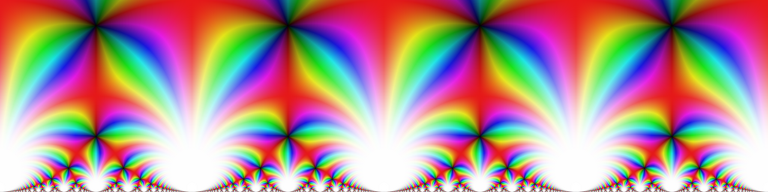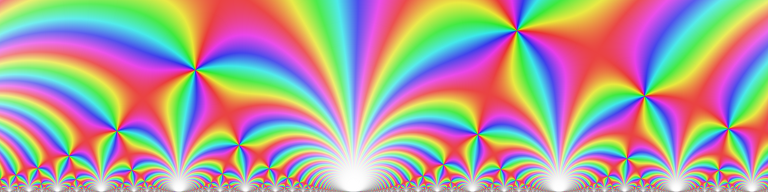# Modular forms in Arb

October 8, 2014

There's a saying (attributed to Martin Eichler) that there are five elementary arithmetical operations: addition, subtraction, multiplication, division, and modular forms. The first four have been well-supported in Arb for some time, and the fifth is just now coming along!

More precisely, the git trunk of Arb now supports arbitrary-precision rigorous-interval evaluation of two famous modular forms: the Dedekind eta function, and Klein's j-invariant. It also supports working with modular transformations and evaluating theta sums. With these building blocks, one can easily implement other modular forms such as Eisenstein series (and indeed, my plan is to provide such functions in the future, as well as elliptic functions).

Geometrically, modular forms are fractals, and thus lend themselves to making pretty pictures. Arb doesn't really have a plotting interface just yet, but it wasn't much work to write a program that converts function values to RGB values and dumps them to a .ppm bitmap, after translating the domain coloring code in mpmath to C (I might clean up this code and put it in the library later):The j-invariant on the complex interval $[-2, 2] + [0, 1]i$. Click for 8192x2048 resolution.The Dedekind eta function on the complex interval $[0, 24] + [0, 1]i$. Click for 24576x1024 resolution.

These high-resolution plots (16 and 25 megapixels respectively) were rendered at 128-bit working precision, and took 5-10 minutes each on a single core (parallelization should be trivial, but I didn't bother as I only had a single-core system when rendering these). Thus, at fairly low precision, Arb manages something like 50,000 function evaluations per second. This is 1-2 orders of magnitude faster than mpmath, and even more when getting close to the real axis (where mpmath currently slows down exponentially).

Indeed, with arbitrary precision and a robust evaluation algorithm comes the possibility to do ultra deep zooms. Below are two plots with a magnification factor of 1 googol $= 10^{100}$:The phase of the Dedekind eta function on the complex interval $[\sqrt{2}, \sqrt{2} + 10^{-101}] + [0, 2.5 \times 10^{-102}]i$. Click for 4096x1024 resolution.The j-invariant on the complex interval $[\sqrt{13}, \sqrt{13} + 10^{-101}] + [0, 2.5 \times 10^{-102}]i$. Click for 4096x1024 resolution.

These 4-megapixel renders took 25 minutes each (on one core) and were done at a working precision of 1024 bits.

Note that there are sampling artifacts if you look closely at these plots. This problem can be avoided with subsampling. In fact, with ball/interval arithmetic, you could generate provably correct plots by evaluating the function on each pixel as a whole (viewed as a rectangle in the complex plane), rather than on infinitesimal sample points, and adaptively subdividing until you can determine the correct averaged color for each pixel. But that is a whole different project!

Modular forms satisfy myriads of functional relations, and tend to have interesting values at special points. For example, as the Wikipedia article about the Dedekind eta function points out, the j-invariant and the eta function are related as $$j(\tau) = \left( \left(\frac{\eta(\tau)}{\eta(2\tau)}\right)^8 + 2^8 \left(\frac{\eta(2\tau)}{\eta(\tau)}\right)^{16} \right)^3$$ and the j-invariant has the special value $$j\left(\frac{1+\sqrt{-163}}{2}\right) = -640320^3$$ which is related to the fact that $e^{\pi\sqrt{163}} \approx 640320^3+743.99999999999925\dots$ is amazingly close to an integer.

Here is a short program that tests these identities. Using ball/interval arithmetic, we expect different formulas for the same mathematical value to output intervals that overlap with each other, and of course also contain the exact value. The program also measures the execution time for the respective function:

#include "acb_modular.h"
#include "profiler.h"

int main()
{
acb_t tau, eta1, eta2, j, t, u, ans;
long prec;

acb_init(tau); acb_init(eta1); acb_init(eta2);
acb_init(j); acb_init(t); acb_init(u); acb_init(ans);

for (prec = 64; prec <= 2e6; prec *= 4)
{
acb_set_si(tau, -163);
acb_sqrt(tau, tau, prec);
acb_mul_2exp_si(tau, tau, -1);

printf("prec = %ld\n", prec);

printf("j:   ");
TIMEIT_START
acb_modular_j(j, tau, prec);
TIMEIT_STOP

printf("eta: ");
TIMEIT_START
acb_modular_eta(eta1, tau, prec);
TIMEIT_STOP

acb_mul_2exp_si(tau, tau, 1);
acb_modular_eta(eta2, tau, prec);
acb_div(t, eta1, eta2, prec);
acb_pow_ui(t, t, 8, prec);
acb_inv(u, t, prec);
acb_mul(u, u, u, prec);
acb_mul_2exp_si(u, u, 8);
acb_pow_ui(t, t, 3, prec);

acb_set_si(ans, -640320);
acb_pow_ui(ans, ans, 3, 64);

printf("overlap: %d, containment: %d\n", acb_overlaps(j, t),
acb_contains(j, ans) && acb_contains(t, ans));

acb_printd(j, 25); printf("\n");
acb_printd(t, 25); printf("\n");
printf("\n");
}

acb_clear(tau); acb_clear(eta1); acb_clear(eta2);
acb_clear(j); acb_clear(t); acb_clear(u); acb_clear(ans);

flint_cleanup();
}


The program outputs the following:

prec = 64
j:   cpu/wall(s): 6.7e-06 7.18e-06
eta: cpu/wall(s): 6.1e-06 6.28e-06
overlap: 1, containment: 1
(-262537412640767998.515625 - 9.583016555401524595206054e-12j)  +/-  (9.25, 3.81e-07j)
(-262537412640767999 - 0.2065797845092608606506644j)  +/-  (78.9, 64.3j)

prec = 256
j:   cpu/wall(s): 1.1e-05 1.24e-05
eta: cpu/wall(s): 1e-05 1.09e-05
overlap: 1, containment: 1
(-262537412640768000 + 6.175588219646460466328569e-68j)  +/-  (1.8e-57, 9.07e-65j)
(-262537412640768000 - 5.265609339283016719999099e-60j)  +/-  (1.26e-56, 1.02e-56j)

prec = 1024
j:   cpu/wall(s): 3e-05 3.14e-05
eta: cpu/wall(s): 3.6e-05 3.66e-05
overlap: 1, containment: 1
(-262537412640768000 - 6.191850416649493269947559e-299j)  +/-  (1.16e-288, 5.89e-296j)
(-262537412640768000 + 2.416555834579265876442343e-290j)  +/-  (7.94e-288, 6.47e-288j)

prec = 4096
j:   cpu/wall(s): 0.00024 0.000248
eta: cpu/wall(s): 0.00036 0.00038
overlap: 1, containment: 1
(-262537412640768000 - 4.985688509409972576666983e-1224j)  +/-  (2e-1213, 1.51e-1220j)
(-262537412640768000 + 8.757024093526885406242355e-1216j)  +/-  (1.34e-1212, 1.09e-1212j)

prec = 16384
j:   cpu/wall(s): 0.0024 0.00249
eta: cpu/wall(s): 0.005 0.0052
overlap: 1, containment: 1
(-262537412640768000 - 5.476336188603508228685505e-4923j)  +/-  (1.75e-4912, 1.37e-4919j)
(-262537412640768000 + 6.406024267686023293746183e-4914j)  +/-  (1.18e-4911, 9.61e-4912j)

prec = 65536
j:   cpu/wall(s): 0.022 0.0235
eta: cpu/wall(s): 0.056 0.0571
overlap: 1, containment: 1
(-262537412640768000 - 4.637927148537148503405785e-19720j)  +/-  (1.04e-19708, 8.59e-19716j)
(-262537412640768000 + 9.510026173830554939511924e-19711j)  +/-  (7e-19708, 5.71e-19708j)

prec = 262144
j:   cpu/wall(s): 0.25 0.266
eta: cpu/wall(s): 0.6 0.616
overlap: 1, containment: 1
(-262537412640768000 + 1.342790082833536950147581e-78904j)  +/-  (1.29e-78893, 1.18e-78900j)
(-262537412640768000 + 1.04058336851956512210966e-78895j)  +/-  (6.82e-78893, 5.56e-78893j)

prec = 1048576
j:   cpu/wall(s): 2.06 2.122
eta: cpu/wall(s): 4.83 4.997
overlap: 1, containment: 1
(-262537412640768000 + 5.517708769240461892678295e-315644j)  +/-  (3.1e-315633, 3.4e-315640j)
(-262537412640768000 + 6.105172555794133440925373e-315635j)  +/-  (2.08e-315632, 1.7e-315632j)


Notably, evaluation of modular forms is very fast at high precision: even a million bits just takes a couple of seconds (this is due to the rapid convergence of the theta function series).